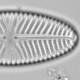## Colour coding points in a graph

Sometimes it is useful to colour code points on a graph according to a categorical variable. There are, as always, several ways to do this. It would be possible to use nested ifelse() statements, but that way lies insanity if there are more than a few levels. An approach that scales better is to have the categorical variable coded as a factor, and use this to subset a vector of colours. Internally, R will call as.numeric() on the factor, so the first level of the factor will get the first value in the vector of colours and so on.

```library(vegan)
data(dune)
data(dune.env)
mod<-cca(dune)

class(dune.env\$Moisture)

colour<-c("red","pink", "lightblue", "blue")
x11(5,5)
par(mar=c(3,3,1,1), mgp=c(1.5,.5,0))
plot(mod, type="n")
points(mod, display="sites", col=colour[dune.env\$Moisture], pch=20)
legend("topright", legend=1:4, col=colour, pch=20, title="Moisture level")
```

Equivalent code could be used to give each level of a categorical variable a different symbol, using the argument pch instead of col.
It is good practice to assign the colours to an object so they can be reused. If you change your mind about which colours you want, you only need edit a single line, reducing the risk of errors.